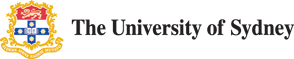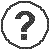# help

School of Chemistry - Chemical and Scientific Calculator
 decimal places 0 1 2 3 4 5 6 7 8 9 10 11 12 13 14 15 Rad Deg Grad

# Converter

 (i) energy units: Convert from: joules (J) calories (Cal) electron volts (eV) cubic centrimetre atmospheres erg atomic units (hartree) Convert to: joules (J) calories (Cal) electron volts (eV) cubic centrimetre atmospheres erg atomic units (hartree)
(ii) energy per mole and molecule and spectroscopic units:
 Convert from: wavelength (nm) wavenumber (1/cm) frequency (Hz) electron volts (eV per molecule) atomic units (hartree per molecule) kilojoules per mole (kJ/mol) Convert to: wavelength (nm) wavenumber (1/cm) frequency (Hz) electron volts (eV per molecule) atomic units (hartree per molecule) kilojoules per mole (kJ/mol)
(iii) pressure:
 Convert from: Pascal (Pa or N/m^2) Bar (bar) Atmospheres (atm) torr or mm of mercury (Torr or mmHg) dyne per cm^2 (dyne/cm^2) pound per square inch (psi) Convert to: Pascal (Pa or N/m^2) Bar (bar) Atmospheres (atm) torr or mm of mercury (Torr or mmHg) dyne per cm^2 (dyne/cm^2) pound per square inch (psi)
(iv) temperature:
 Convert from: Kelvins (K) Celsius (' C) Fahrenheit (' F) Réaumur (' Réaumur) Rankine (' Rankine) Convert to: Kelvins (K) Celsius (' C) Fahrenheit (' F) Réaumur (' Réaumur) Rankine (' Rankine)
(v) length:
 Convert from: metres (m) picometres (pm) nanometres (nm) angstroms (A) Bohrs (au) inches feet yards miles Convert to: metres (m) picometres (pm) nanometres (nm) angstroms (A) Bohrs (au) inches feet yards miles
(vi) area:
 Convert from: square metre (m^2) square decimetre (dm^2) square centimetre (cm^2) square angstroms (A^2) square inches square feet square yards Convert to: square metre (m^2) square decimetre (dm^2) square centimetre (cm^2) square angstroms (A^2) square inches square feet square yards
(vii) volume:
 Convert from: cubic metre (m^3) cubic decimetre (dm^3) cubic centimetre (cm^3) litre (l) centilitre (cl) millimetre (ml) cubic angstroms (A^3) cubic inches cubic feet cubic yards Convert from: Convert to: cubic metre (m^3) cubic decimetre (dm^3) cubic centimetre (cm^3) litre (l) centilitre (cl) millimetre (ml) cubic angstroms (A^3) cubic inches cubic feet cubic yards
(viii) mass:
 Convert from: kilograms (kg) grams (g) metric tonnes (t) atomic mass units (amu) ounces pounds stones tons Convert to: kilograms (kg) grams (g) metric tonnes (t) atomic mass units (amu) ounces pounds stones tons